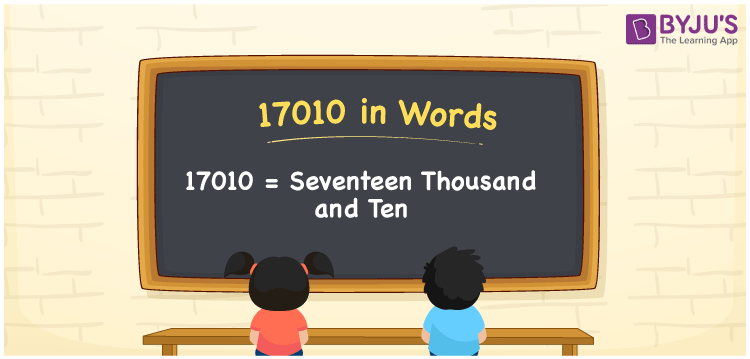# 17010 in Words

18500 in words is Seventeen Thousand and Ten. For example, if you have 17010 rupees in your account, then you can write, “I have Seventeen Thousand and Ten rupees in my account”. We know that the number name of any number can be written using the ones, tens, hundreds and thousands place of a number. Therefore, the place value chart is essential to write 17010 in words. 17010 is a cardinal number as it shows a specific quantity.

 17010 in words Seventeen Thousand and Ten Seventeen Thousand and Ten in Numbers 17010

## 17010 in English Words## How to Write 17010 in Words?

We can convert 17010 to words using a place value chart. This can be done as follows. The number 17010 has 5 digits, so let’s make a chart that shows the place value up to 5 digits.

 Ten thousands Thousands Hundreds Tens Ones 1 7 0 1 0

Thus, we can write the expanded form as:

1 × Ten thousand + 7 × Thousand + 0 × Hundred + 1 × Ten + 0 × One

= 1 × 10000 + 7 × 1000 + 0 × 100 + 1 × 10 + 0 × 1

= 17010

= Seventeen Thousand and Ten

17010 is the natural number that is succeeded by 17009 and preceded by 17011.

17010 in words – Seventeen Thousand and Ten

Is 17010 an odd number? – No.

Is 17010 an even number? – Yes

Is 17010 a perfect square number? – No

Is 17010 a perfect cube number? – No

Is 17010 a prime number? – No

Is 17010 a composite number? – Yes

## Frequently Asked Questions on 17010 in Words

Q1

### How to write 17010 in words?

17010 in English is written as “Seventeen Thousand and Ten”.
Q2

### Is the number 17010 an odd number?

No, the number 17010 is not an odd number.
Q3

### Is 17010 a composite number?

Yes, 17010 is a composite number.